# Rectangle

For the record label, see Rectangle (label).
RectangleRectangle
Edges and vertices 4
Schläfli symbol { } × { }
Coxeter diagramSymmetry group Dihedral (D2), , (*22), order 4
Dual polygon rhombus
Properties convex, isogonal, cyclic Opposite angles and sides are congruent

In Euclidean plane geometry, a rectangle is a quadrilateral with four right angles. It can also be defined as an equiangular quadrilateral, since equiangular means that all of its angles are equal (360°/4 = 90°). It can also be defined as a parallelogram containing a right angle. A rectangle with four sides of equal length is a square. The term oblong is occasionally used to refer to a non-square rectangle. A rectangle with vertices ABCD would be denoted as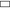ABCD.

The word rectangle comes from the Latin rectangulus, which is a combination of rectus (right) and angulus (angle).

A crossed rectangle is a crossed (self-intersecting) quadrilateral which consists of two opposite sides of a rectangle along with the two diagonals. It is a special case of an antiparallelogram, and its angles are not right angles. Other geometries, such as spherical, elliptic, and hyperbolic, have so-called rectangles with opposite sides equal in length and equal angles that are not right angles.

Rectangles are involved in many tiling problems, such as tiling the plane by rectangles or tiling a rectangle by polygons.

## Characterizations

A convex quadrilateral is a rectangle if and only if it is any one of the following:

• a quadrilateral with four right angles
• a parallelogram with at least one right angle
• a parallelogram with diagonals of equal length
• a parallelogram ABCD where triangles ABD and DCA are congruent
• a convex quadrilateral with successive sides a, b, c, d whose area is.:fn.1
• a convex quadrilateral with successive sides a, b, c, d whose area is## Classification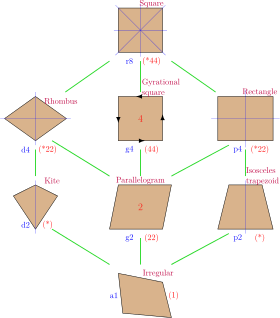A rectangle is a special case of both parallelogram and trapezoid. A square is a special case of a rectangle.

A rectangle is a special case of a parallelogram in which each pair of adjacent sides is perpendicular.

A parallelogram is a special case of a trapezium (known as a trapezoid in North America) in which both pairs of opposite sides are parallel and equal in length.

A trapezium is a convex quadrilateral which has at least one pair of parallel opposite sides.

• Simple: The boundary does not cross itself.
• Star-shaped: The whole interior is visible from a single point, without crossing any edge.

### Alternative hierarchy

De Villiers defines a rectangle more generally as any quadrilateral with axes of symmetry through each pair of opposite sides. This definition includes both right-angled rectangles and crossed rectangles. Each has an axis of symmetry parallel to and equidistant from a pair of opposite sides, and another which is the perpendicular bisector of those sides, but, in the case of the crossed rectangle, the first axis is not an axis of symmetry for either side that it bisects.

Quadrilaterals with two axes of symmetry, each through a pair of opposite sides, belong to the larger class of quadrilaterals with at least one axis of symmetry through a pair of opposite sides. These quadrilaterals comprise isosceles trapezia and crossed isosceles trapezia (crossed quadrilaterals with the same vertex arrangement as isosceles trapezia).

## Properties

### Symmetry

A rectangle is cyclic: all corners lie on a single circle.

It is equiangular: all its corner angles are equal (each of 90 degrees).

It is isogonal or vertex-transitive: all corners lie within the same symmetry orbit.

It has two lines of reflectional symmetry and rotational symmetry of order 2 (through 180°).

### Rectangle-rhombus duality

The dual polygon of a rectangle is a rhombus, as shown in the table below.

Rectangle Rhombus
All angles are equal. All sides are equal.
Alternate sides are equal. Alternate angles are equal.
Its centre is equidistant from its vertices, hence it has a circumcircle. Its centre is equidistant from its sides, hence it has an incircle.
Its axes of symmetry bisect opposite sides. Its axes of symmetry bisect opposite angles.
Diagonals are equal in length. Diagonals intersect at equal angles.
• The figure formed by joining, in order, the midpoints of the sides of a rectangle is a rhombus and vice versa.

### Miscellaneous

The two diagonals are equal in length and bisect each other. Every quadrilateral with both these properties is a rectangle.

A rectangle is rectilinear: its sides meet at right angles.

A rectangle in the plane can be defined by five independent degrees of freedom consisting, for example, of three for position (comprising two of translation and one of rotation), one for shape (aspect ratio), and one for overall size (area).

Two rectangles, neither of which will fit inside the other, are said to be incomparable.

## Formulae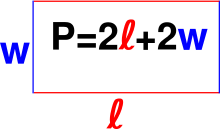The formula for the perimeter of a rectangle.

If a rectangle has lengthand width• it has area,
• it has perimeter,
• each diagonal has length,
• and when, the rectangle is a square.

## Theorems

The isoperimetric theorem for rectangles states that among all rectangles of a given perimeter, the square has the largest area.

The midpoints of the sides of any quadrilateral with perpendicular diagonals form a rectangle.

A parallelogram with equal diagonals is a rectangle.

The Japanese theorem for cyclic quadrilaterals states that the incentres of the four triangles determined by the vertices of a cyclic quadrilateral taken three at a time form a rectangle.

The British flag theorem states that with vertices denoted A, B, C, and D, for any point P on the same plane of a rectangle:For every convex body C in the plane, we can inscribe a rectangle r in C such that a homothetic copy R of r is circumscribed about C and the positive homothety ratio is at most 2 and.

## Crossed rectangles

A crossed (self-intersecting) quadrilateral consists of two opposite sides of a non-self-intersecting quadrilateral along with the two diagonals. Similarly, a crossed rectangle is a crossed quadrilateral which consists of two opposite sides of a rectangle along with the two diagonals. It has the same vertex arrangement as the rectangle. It appears as two identical triangles with a common vertex, but the geometric intersection is not considered a vertex.

A crossed quadrilateral is sometimes likened to a bow tie or butterfly. A three-dimensional rectangular wire frame that is twisted can take the shape of a bow tie. A crossed rectangle is sometimes called an "angular eight".

The interior of a crossed rectangle can have a polygon density of ±1 in each triangle, dependent upon the winding orientation as clockwise or counterclockwise.

A crossed rectangle is not equiangular. The sum of its interior angles (two acute and two reflex), as with any crossed quadrilateral, is 720°.

A rectangle and a crossed rectangle are quadrilaterals with the following properties in common:

• Opposite sides are equal in length.
• The two diagonals are equal in length.
• It has two lines of reflectional symmetry and rotational symmetry of order 2 (through 180°).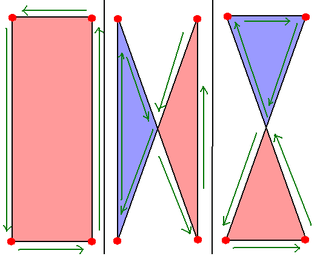## Other rectangles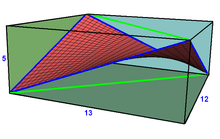A saddle rectangle has 4 nonplanar vertices, alternated from vertices of a cuboid, with a unique minimal surface interior defined as a linear combination of the four vertices, creating a saddle surface. This example shows 4 blue edges of the rectangle, and two green diagonals, all being diagonal of the cuboid rectangular faces.

In spherical geometry, a spherical rectangle is a figure whose four edges are great circle arcs which meet at equal angles greater than 90°. Opposite arcs are equal in length. The surface of a sphere in Euclidean solid geometry is a non-Euclidean surface in the sense of elliptic geometry. Spherical geometry is the simplest form of elliptic geometry.

In elliptic geometry, an elliptic rectangle is a figure in the elliptic plane whose four edges are elliptic arcs which meet at equal angles greater than 90°. Opposite arcs are equal in length.

In hyperbolic geometry, a hyperbolic rectangle is a figure in the hyperbolic plane whose four edges are hyperbolic arcs which meet at equal angles less than 90°. Opposite arcs are equal in length.

## Tessellations

The rectangle is used in many periodic tessellation patterns, in brickwork, for example, these tilings: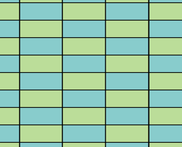Stacked bond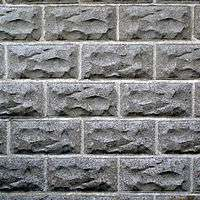Running bond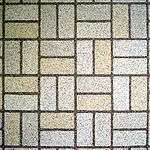Basket weave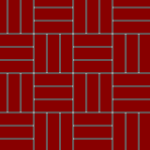Basket weave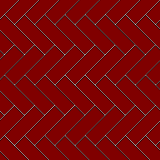Herringbone pattern

## Squared, perfect, and other tiled rectangles

A rectangle tiled by squares, rectangles, or triangles is said to be a "squared", "rectangled", or "triangulated" (or "triangled") rectangle respectively. The tiled rectangle is perfect if the tiles are similar and finite in number and no two tiles are the same size. If two such tiles are the same size, the tiling is imperfect. In a perfect (or imperfect) triangled rectangle the triangles must be right triangles.

A rectangle has commensurable sides if and only if it is tileable by a finite number of unequal squares. The same is true if the tiles are unequal isosceles right triangles.

The tilings of rectangles by other tiles which have attracted the most attention are those by congruent non-rectangular polyominoes, allowing all rotations and reflections. There are also tilings by congruent polyaboloes.

## References

1. http://www.cimt.plymouth.ac.uk/resources/topics/art002.pdf
2. Definition of Oblong. Mathsisfun.com. Retrieved 2011-11-13.
3. Oblong – Geometry – Math Dictionary. Icoachmath.com. Retrieved 2011-11-13.
4. Coxeter, Harold Scott MacDonald; Longuet-Higgins, M.S.; Miller, J.C.P. (1954). "Uniform polyhedra". Philosophical Transactions of the Royal Society of London. Series A. Mathematical and Physical Sciences. The Royal Society. 246 (916): 401–450. doi:10.1098/rsta.1954.0003. ISSN 0080-4614. JSTOR 91532. MR 0062446.
5. Zalman Usiskin and Jennifer Griffin, "The Classification of Quadrilaterals. A Study of Definition", Information Age Publishing, 2008, pp. 34–36 ISBN 1-59311-695-0.
6. Owen Byer; Felix Lazebnik; Deirdre L. Smeltzer (19 August 2010). Methods for Euclidean Geometry. MAA. pp. 53–. ISBN 978-0-88385-763-2. Retrieved 2011-11-13.
7. Martin Josefsson, "Five Proofs of an Area Characterization of Rectangles", Forum Geometricorum 13 (2013) 17–21.
8. An Extended Classification of Quadrilaterals (An excerpt from De Villiers, M. 1996. Some Adventures in Euclidean Geometry. University of Durban-Westville.)
9. de Villiers, Michael, "Generalizing Van Aubel Using Duality", Mathematics Magazine 73 (4), Oct. 2000, pp. 303-307.
10. Cyclic Quadrilateral Incentre-Rectangle with interactive animation illustrating a rectangle that becomes a 'crossed rectangle', making a good case for regarding a 'crossed rectangle' as a type of rectangle.
11. Hall, Leon M. & Robert P. Roe (1998). "An Unexpected Maximum in a Family of Rectangles" (PDF). Mathematics Magazine. 71 (4): 285–291. JSTOR 2690700.
12. Lassak, M. (1993). "Approximation of convex bodies by rectangles". Geometriae Dedicata. 47: 111. doi:10.1007/BF01263495.
13. Stars: A Second Look. (PDF). Retrieved 2011-11-13.
14. R.L. Brooks, C.A.B. Smith, A.H. Stone and W.T. Tutte (1940). "The dissection of rectangles into squares". Duke Math. J. 7 (1): 312–340. doi:10.1215/S0012-7094-40-00718-9.
15. J.D. Skinner II, C.A.B. Smith and W.T. Tutte (November 2000). "On the Dissection of Rectangles into Right-Angled Isosceles Triangles". J. Combinatorial Theory Series B. 80 (2): 277–319. doi:10.1006/jctb.2000.1987.
16. R. Sprague (1940). "Ũber die Zerlegung von Rechtecken in lauter verschiedene Quadrate". J. fũr die reine und angewandte Mathematik. 182: 60–64.Wikimedia Commons has media related to Rectangles.